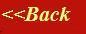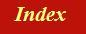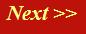#### Tutorials:

TEM alignment

STEM alignment

Wave interference

#### Research:

Diffractive imaginging

## Crystallography and diffraction: a very simple introduction

We can use our concept of the corkscrew function and phase threads to construct a picture of diffraction from a three-dimensional array of atoms. What follows applies to X-ray diffraction and (ignoring to begin with the very important effects of multiple scattering) electron diffraction.

Consider a source of X-ray or electrons incident upon an atom. At the atom, the radiation is scattered: all obstacles, whether objects or apertures (like the harbour wall) diffract waves. We can think of this in terms of our phase threads as follows. The incident wave is represented by a phase thread, coming towards the atom from a certain direction. The scattered wave is also a phase thread, coming away from the atom at a different angle, like this:Now, in fact, the phase of the phase thread (the scattered wave) is usually altered by the act of being scattered by the atom, but in what follows this is a minor consideration, because we are only interested in interfering scattered waves, all of which have (usually) suffered a similar phase change. In other words, we can think of the scattered phase thread setting off with the same phase as the incident beam. We can model the scattering from an atom as simply a phase thread being threaded through a point in space, like the eye of a needle, at the exact position of the atom, as shown below: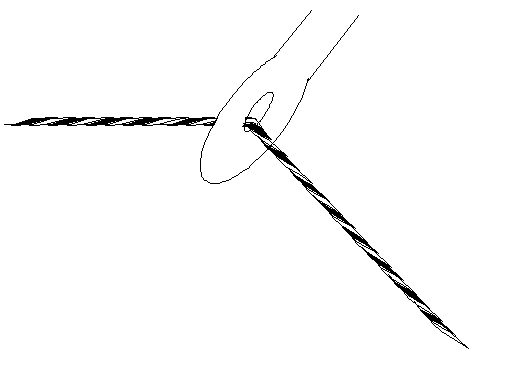For two atoms, we have to draw two phase threads. The incoming phase threads are in phase, because they represent a plane wave incident upon the atoms. The scattered phase threads are parallel to one another, but of course will have a relative phase difference between them according to the exact position of the two atoms, like this: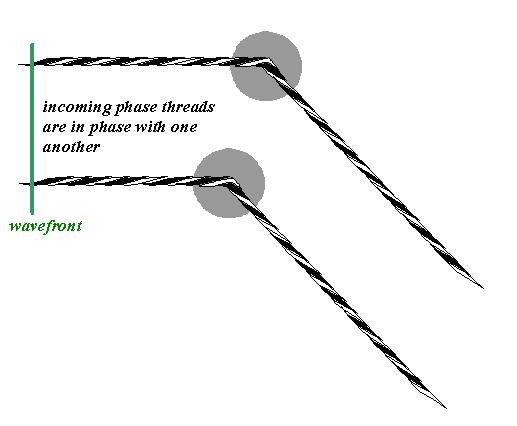In the far-field (that is, the Fraunhofer diffraction plane – we are assuming here that the source and the detector are far enough away from the atoms to satisfy the Fraunhofer condition), the scattered waves interfere, according to the usual rule: their complex amplitudes add and the intensity measured is the square of the resulting magnitude of the complex sum.

We should say that heavy atoms (atoms with a large number of electrons) tend to scatter X-rays more strongly than light atoms. In the case of electron diffraction, it is the atomic potential (its sharpness in space) that defines the scattering amplitude. Suffice it to say, different elements scatter with different amplitudes (this also varies as a function of the total angle of scatter). So if our atoms have different scattering strengths, then the scattered waves must have associated with them different magnitudes. So, thinking of three atoms, each having different scattering amplitudes, the phase threads might look like this: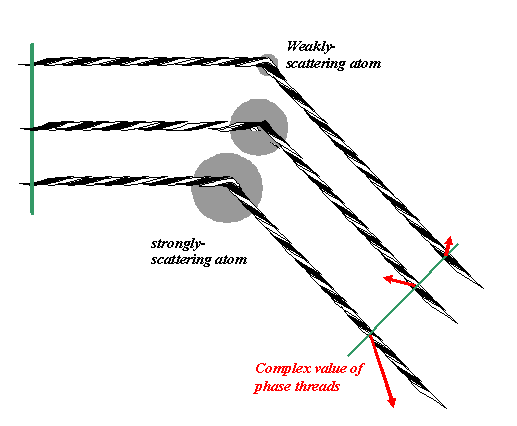The green line is perpendicular to the phase threads. The relative phase (direction of the red arrows) depends on the relative position of the phase threads where they cross the green line. The lengths of these red arrows correspond to the magnitude (modulus) of the wave scattered from the different atoms.

The corresponding addition of all threads in the complex plane looks like this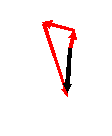where the black pointer is the resultant amplitude of the three waves when they meet up in the Fraunhofer diffraction plane.

In a real diffraction experiment, we don’t have three atoms; we have many billions of atoms involved (unless we are thinking rather specialised types of electron diffraction where it is possible to isolate only a few atoms). Anyway, I’m going to draw ten atoms, because that’s all I can be bothered to do: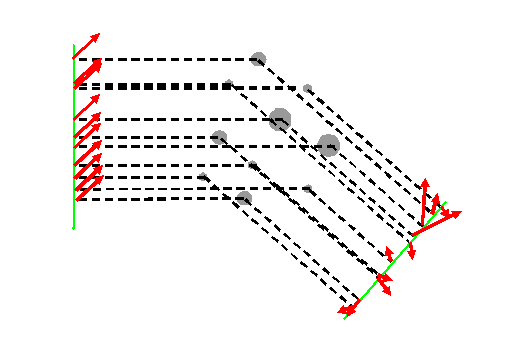On the vertical green line we plot the complex values (the red pointers) of the incident phase threads. The phases of the scattered threads, heading towards a detector in the far-field somewhere a very long way down to the bottom right of diagram, are principally determined by geometry – the positions of the atoms. The green line on the right of the diagram is just an arbitrary plane drawn perpendicular to the scattered phase threads. The actual phase of each thread over this plane depends on the exact position of where the green line intersects the particular thread, but because ultimately we are only interested in the intensity of the resultant of the addition of the complex values (red pointers) associated with these threads, only their relative phases matter. No matter how far away is the detector plane, these relative phases will be preserved until the phase threads meet up and interfere with each other.

At the detector, the scattered waves add as before – there are just many more of them.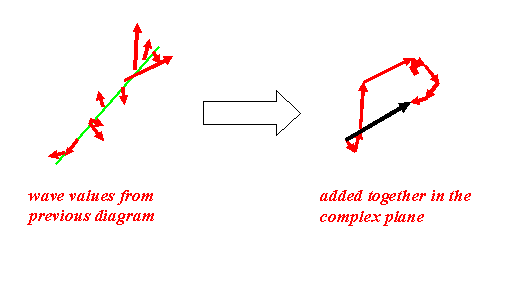Remember that the intensity recorded is the length of the resultant (the length of the black vector) squared.

And…er…that’s it! This is really the whole story of single-scattering diffraction.

### Then why is crystallography so complicated?

Being able to work out the diffracted intensity at a certain angle using our phase thread model is one thing (conceptually, a very easy thing); being able to infer from the diffracted intensity the structure of the object concerned is quite another discipline.

Now it so happens that most elements, inorganic compounds and very many organic molecules can be made to form regular, periodic arrays of atoms or molecules: that is, they form crystals. Crystals have their own mathematical structure, quite independent of how we measure their physical manifestation using diffraction. The mathematics of the symmetry of three-dimensional, periodic space has a complicated structure, described by symmetry groups. When you perform certain operations on a crystal structure, such as translations, rotations, reflections through planes or points, etc, then the object under consideration looks identical to what it did before the operation: it has certain symmetries.

Crystallographers catalogue all these various structures according to rather abstract symmetry groups: there are 32 point groups and 230 space groups. This is a mathematical truth, but one that happens to get expressed in nature when atoms of various sizes and chemical bonding affinities are forced together in three-dimensional space. A lot of the complication of crystallography derives from this symmetry classification.

Of course, it goes without saying that working out the structure matter from a diffraction pattern alone would be impossible (well, not quite impossible – but this another story at the cutting edge of present-day research) if it was not for the fact that so many materials do form crystals. However, do not let the subtleties of crystallography impede your understanding of diffraction.

### What we actually have to understand:

1) That ‘reciprocal space’ is the Fourier transform of real space.

2) The ‘reciprocal lattice’ is the Fourier transform of a real space lattice. We already know how this works in one dimension (the one dimensional grating).

3) The scattering vector ‘K’, which we first introduced on the previous page, is intimately connected with how ‘reciprocal space’ is related to the incident (illumination) plane waves (the incident beam) and the plane waves which are scattered by the object (the scattered beam). This can be derived using Bragg’s Law in real space or the Ewald sphere in reciprocal space.

4) That electron diffraction is usually dominated by multiple scattering, but that the models in (1)-(3) above are nevertheless a good starting point for thinking about what happens in multiple-scattering.

In order to tackle these points, we must first ensure that we understand three-dimensional Fourier transforms and how K, the scattering vector, relates to the incident and scattered beams (next page). We will then rehearse Bragg’s law in terms of the phase threads before we put all these ideas together.